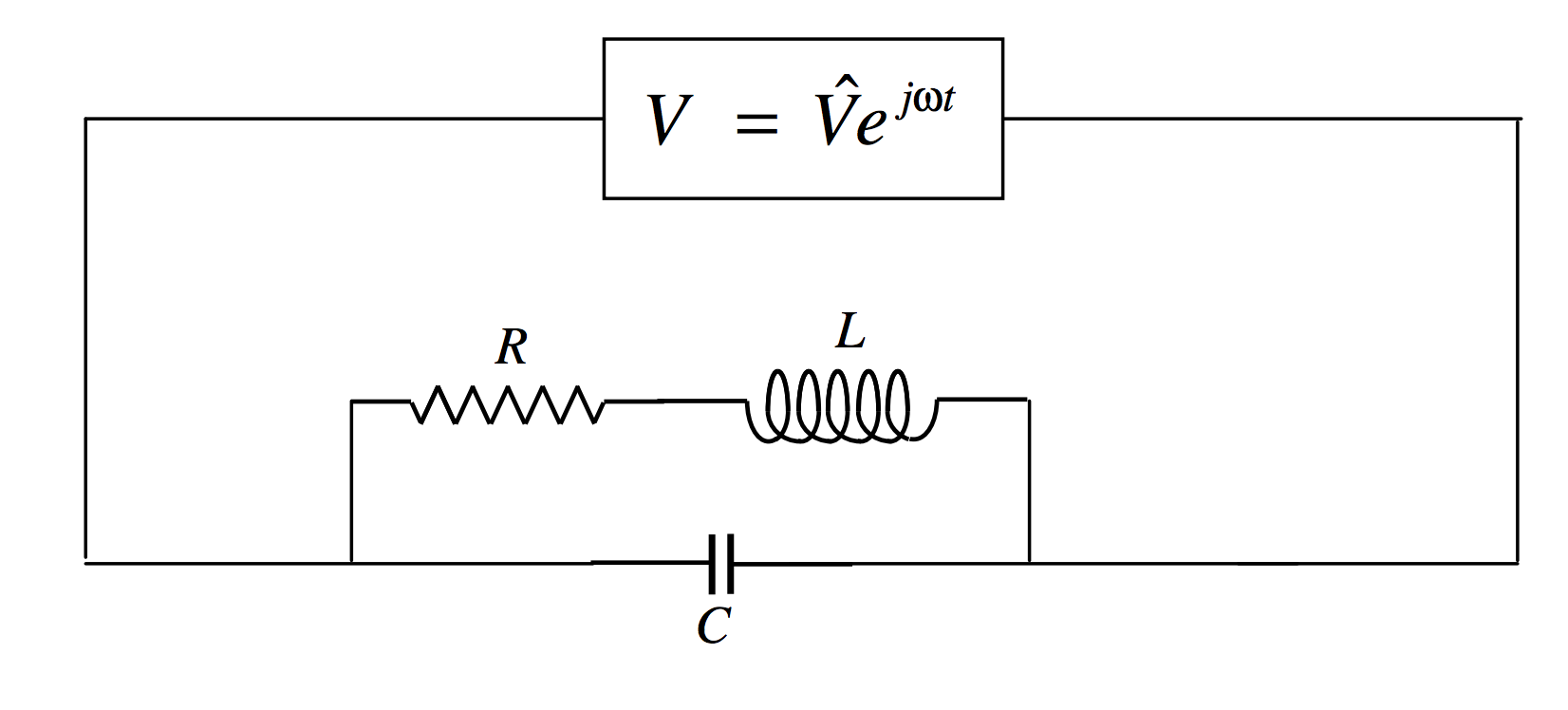$$\require{cancel}$$

# 13.8: The RLC Parallel Rejector Circuit

•• Contributed by Jeremy Tatum
• Emeritus Professor (Physics & Astronomy) at University of Victoria

In the circuit below, the magnitude of the admittance is least for certain values of the parameters. When you tune a radio set, you are changing the overlap area (and hence the capacitance) of the plates of a variable air-spaced capacitor so that the admittance is a minimum for a given frequency, so as to ensure the highest potential difference across the circuit. This resonance, as we shall see, does not occur for an angular frequency of exactly $$1/\sqrt{LC}$$, but at an angular frequency that is approximately this if the resistance is small.$\label{13.8.1}Y=jC\omega+\frac{1}{R+jL\omega}.$

After some routine algebra (multiply top and bottom by the conjugate; then collect real and imaginary parts), this becomes

$\label{13.8.2}Y=\frac{R+j\omega (L^2C\omega^2 +R^2C-L)}{R^2+L^2\omega^2}.$

The magnitude of the admittance is least when the susceptance is zero, which occurs at an angular frequency of

$\label{13.8.3}\omega_0^2=\frac{1}{LC}-\frac{R^2}{L^2}.$

If $$R << \sqrt{L/C}$$ this is approximately $$1/\sqrt{LC}$$.